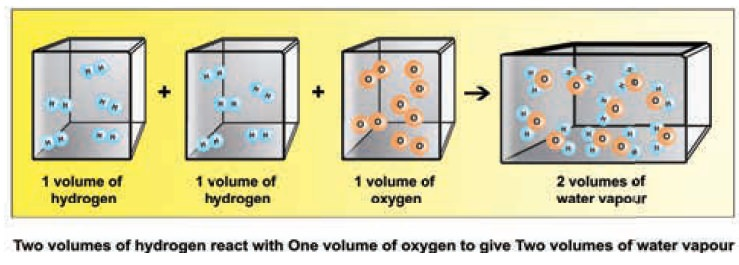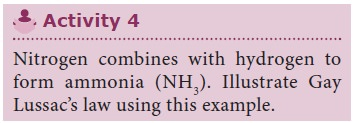Home | | Science 9th std | Law of Reciprocal Proportions

# Law of Reciprocal Proportions

The law of reciprocal proportions was proposed by Jeremias Ritcher in 1792.

Law of Reciprocal Proportions

The law of reciprocal proportions was proposed by Jeremias Ritcher in 1792.It states that, â€śIf two different elements combine separately with the same weight of a third element, the ratios of the masses in which they do so are either the same or a simple multiple of the mass ratio in which they combine.â€ť

Let us study the following example Here carbon combines with hydrogen and oxygen to form Methane (CH4) and CO2 (carbon dioxide) respectively. Hydrogen and oxygen combine to form water.It is seen that in CH4 the ratio of masses C : H = 3:1

In CO2 the ratio of masses of C : O = 3:8

Here hydrogen and oxygen combine with the same mass of carbon. They also combine with each other to form water (H2O)

What is the ratio of masses of H and O in H2O?

It is 2: 16 or 1:8 which is same as 4:32, which is the ratio of the different masses of hydrogen and oxygen combining with the same mass of carbon.

This illustrates the law of reciprocal proportions.

Let us consider one more example.

Sulphur combines with oxygen to form sulphur dioxide, carbon combines with oxygen to form carbon dioxide and carbon combines with sulphur to form carbon disulphideThe ratio of masses of carbon and sulphur which combine with fixed mass (32 parts) of oxygen is

12:32 or 3:8         â€¦â€¦â€¦â€¦..(1)

In CS2 ratio of masses of carbon and sulphur is in the ratio

12:64 or 3:16          â€¦â€¦â€¦â€¦â€¦â€¦â€¦(2)### Solved problem

Hydrogen sulphide (H2 S) contains 94.11% sulphur, water (H2O) contains 11.11% hydrogen and sulphur dioxide (SO2) contains 50% of oxygen. Show that the results are in agreement with the law of reciprocal proportions.

Solution

In 100g of water, the weight of hydrogen =  11.11 g

The weight of oxygen = 100 â€“ 11.11 = 88.89 g

In 100g of sulphur dioxide, the weight of sulphur = 50 g

Weight of oxygen = 100 â€“ 50 = 50 g

The ratio between the weight of oxygen and Hydrogen is 88.89:11.11 i.e. 8:1 (1)

In hydrogen sulphide, the weight of sulphur = 94.11 g

The weight of hydrogen = 100 â€“ 94.11 = 5.89 g

The ratio between the weight of sulphur and hydrogen is 94.11: 5.89 ie. 16: 1 â€¦ (2)

The two ratios 1 and 2 are related as 8/1: 16/1 (or) 1 : 2

These are simple multiples of each other. The ratio between the weight of sulphur (32) and oxygen (16) which combine separately with the weight of Hydrogen (2) supports the law of reciprocal proportions.

## 1. Gay Lussacâ€™s law ofCombining VolumesStep 1: Hydrogen combines with oxygen to form water (word equation)

Step2: H2 + Â˝ O2â†’ H2O (skeletal equation)

Step3: 2H2(g)+ O2(g)â†’ 2H20 (g) (balanced equation)

(2 Volumes) + (1 Volume)â†’(2 Volumes) (2:1:2)

i.e. two volumes of hydrogen react with 1 volume of oxygen to form two volumes of water vapour. i.e. the ratio by volume which gases bears is 2:1:2 which is a simple whole number ratio.

It follows that at a given temperature and pressure the volumes of all gaseous reactants and products bear a simple whole number ratio to each other.Let us consider one more example:

Step 1: Hydrogen combines with chlorine to form hydrogen chloridei.e. one volume of hydrogen reacts with one volume of chlorine to form two volumes of HCl gas. i.e. the ratio by volume which gases bears is 1:1:2 which is a simple whole number ratio.### Solved Problem

Methane burns in oxygen to form carbon dioxide and water vapour as given by the equation

CH4(g) + 2O2(g) â†’ CO2(g) + 2H2O(g)

Calculate: (i) the volume of oxygen needed to burn completely 50 cm3 of methane and the volume of carbon dioxide formed in this case.

Solution:Tags : Atomic Structure | Chemistry , 9th Science : Atomic Structure
Study Material, Lecturing Notes, Assignment, Reference, Wiki description explanation, brief detail
9th Science : Atomic Structure : Law of Reciprocal Proportions | Atomic Structure | Chemistry